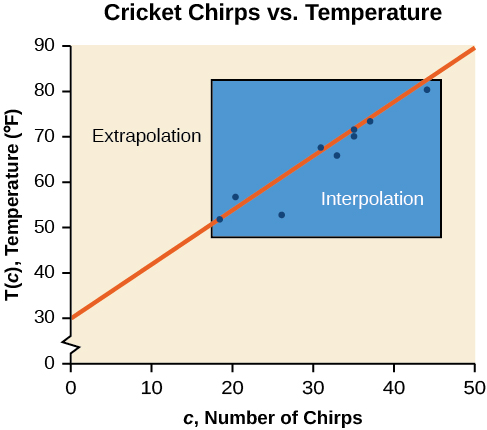# 2.4 Fitting linear models to data  (Page 2/14)

 Page 2 / 14

[link] compares the two processes for the cricket-chirp data addressed in [link] . We can see that interpolation would occur if we used our model to predict temperature when the values for chirps are between 18.5 and 44. Extrapolation would occur if we used our model to predict temperature when the values for chirps are less than 18.5 or greater than 44.

There is a difference between making predictions inside the domain and range of values for which we have data and outside that domain and range. Predicting a value outside of the domain and range has its limitations. When our model no longer applies after a certain point, it is sometimes called model breakdown    . For example, predicting a cost function for a period of two years may involve examining the data where the input is the time in years and the output is the cost. But if we try to extrapolate a cost when $x=50,$ that is in 50 years, the model would not apply because we could not account for factors fifty years in the future.Interpolation occurs within the domain and range of the provided data whereas extrapolation occurs outside.

## Interpolation and extrapolation

Different methods of making predictions are used to analyze data.

• The method of interpolation    involves predicting a value inside the domain and/or range of the data.
• The method of extrapolation    involves predicting a value outside the domain and/or range of the data.
• Model breakdown occurs at the point when the model no longer applies.

## Understanding interpolation and extrapolation

Use the cricket data from [link] to answer the following questions:

1. Would predicting the temperature when crickets are chirping 30 times in 15 seconds be interpolation or extrapolation? Make the prediction, and discuss whether it is reasonable.
2. Would predicting the number of chirps crickets will make at 40 degrees be interpolation or extrapolation? Make the prediction, and discuss whether it is reasonable.
1. The number of chirps in the data provided varied from 18.5 to 44. A prediction at 30 chirps per 15 seconds is inside the domain of our data, so would be interpolation. Using our model:

Based on the data we have, this value seems reasonable.
2. The temperature values varied from 52 to 80.5. Predicting the number of chirps at 40 degrees is extrapolation because 40 is outside the range of our data. Using our model:

We can compare the regions of interpolation and extrapolation using [link] .

According to the data from [link] , what temperature can we predict it is if we counted 20 chirps in 15 seconds?

$54°\text{F}$

## Finding the line of best fit using a graphing utility

While eyeballing a line works reasonably well, there are statistical techniques for fitting a line to data that minimize the differences between the line and data values Technically, the method minimizes the sum of the squared differences in the vertical direction between the line and the data values. . One such technique is called least squares regression    and can be computed by many graphing calculators, spreadsheet software, statistical software, and many web-based calculators For example, http://www.shodor.org/unchem/math/lls/leastsq.html . Least squares regression is one means to determine the line that best fits the data, and here we will refer to this method as linear regression.

#### Questions & Answers

how fast can i understand functions without much difficulty
Joe Reply
what is set?
Kelvin Reply
a colony of bacteria is growing exponentially doubling in size every 100 minutes. how much minutes will it take for the colony of bacteria to triple in size
Divya Reply
I got 300 minutes. is it right?
Patience
no. should be about 150 minutes.
Jason
It should be 158.5 minutes.
Mr
ok, thanks
Patience
100•3=300 300=50•2^x 6=2^x x=log_2(6) =2.5849625 so, 300=50•2^2.5849625 and, so, the # of bacteria will double every (100•2.5849625) = 258.49625 minutes
Thomas
what is the importance knowing the graph of circular functions?
Arabella Reply
can get some help basic precalculus
ismail Reply
What do you need help with?
Andrew
how to convert general to standard form with not perfect trinomial
Camalia Reply
can get some help inverse function
ismail
Rectangle coordinate
Asma Reply
how to find for x
Jhon Reply
it depends on the equation
Robert
yeah, it does. why do we attempt to gain all of them one side or the other?
Melissa
whats a domain
mike Reply
The domain of a function is the set of all input on which the function is defined. For example all real numbers are the Domain of any Polynomial function.
Spiro
Spiro; thanks for putting it out there like that, 😁
Melissa
foci (–7,–17) and (–7,17), the absolute value of the differenceof the distances of any point from the foci is 24.
Churlene Reply
difference between calculus and pre calculus?
Asma Reply
give me an example of a problem so that I can practice answering
Jenefa Reply
x³+y³+z³=42
Robert
dont forget the cube in each variable ;)
Robert
of she solves that, well ... then she has a lot of computational force under her command ....
Walter
what is a function?
CJ Reply
I want to learn about the law of exponent
Quera Reply
explain this
Hinderson Reply

### Read also:

#### Get Jobilize Job Search Mobile App in your pocket Now!

Source:  OpenStax, Precalculus. OpenStax CNX. Jan 19, 2016 Download for free at https://legacy.cnx.org/content/col11667/1.6
Google Play and the Google Play logo are trademarks of Google Inc.

Notification Switch

Would you like to follow the 'Precalculus' conversation and receive update notifications?ByByBy OpenStaxBy Cath YuBy Zarina ChocolateBy OpenStaxBy Saylor FoundationByByBy OpenStaxBy Cath YuBy Gerr Zen